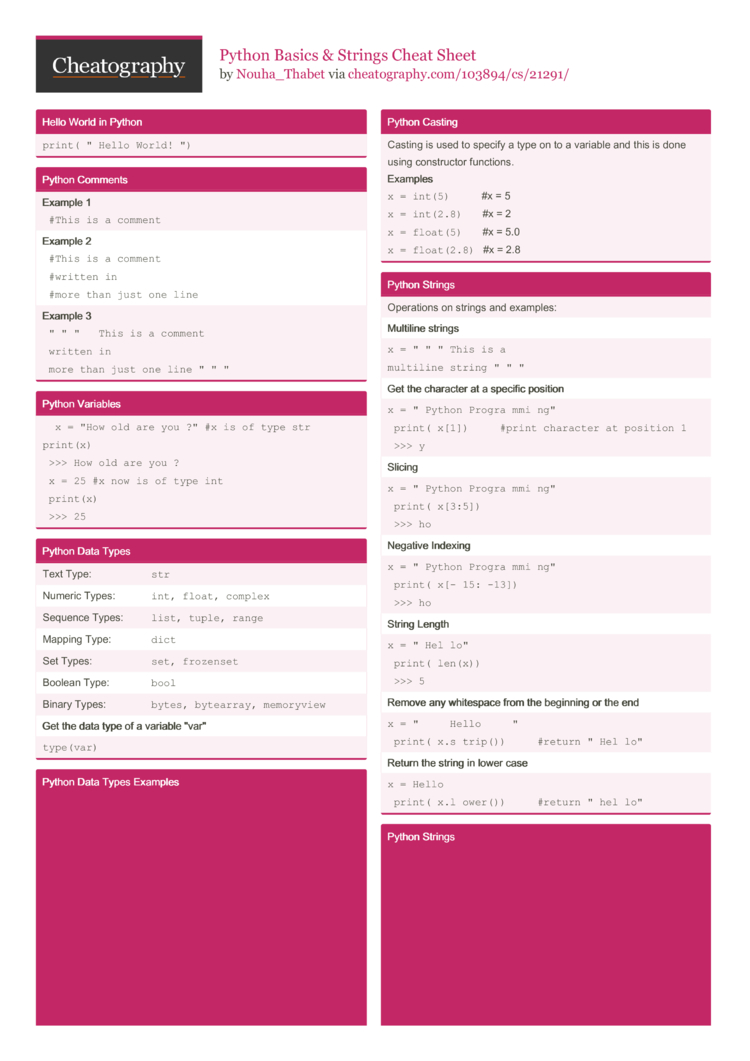# Python Basics & Strings Cheat Sheet by Nouha_Thabet

### Hello World in Python

 `print­("Hello World!­")`

 Example 1 ` #This is a comment ` Example 2 ` #This is a comment #written in #more than just one line ` Example 3 ` "­"­" This is a comment written in more than just one line "­"­" `

### Python Variables

 ` x = "How old are you ?" #x is of type str print(x) >>> How old are you ?` ` x = 25 #x now is of type int print(x) >>> 25 `

### Python Data Types

 Text Type: `str` Numeric Types: `int, float, complex` Sequence Types: `list, tuple, range` Mapping Type: `dict` Set Types: `set, frozenset` Boolean Type: `bool` Binary Types: `bytes, bytearray, memory­view` Get the data type of a variable "­var­" `type(­var)`

### Python Data Types Examples

 Exam­ple Data Type `x = "­­Co­lor­"` `list` `x = 1` `int` `x = 1.2` `float` `x = 2j` `complex` `x = ["Bl­­ue­"­,­"­­Red­­"­,­"­Ye­­llo­­w"]` `list` `x = ("Bl­ue",­"­Red­"­,"Ye­llo­w")` `tuple` `x = range(8)` `range` `x={"Ag­e":2­5,"H­eig­ht":­1.72}` `dict` `x = {"Pi­nk",­"­Red­"}` `set` `x = frozen­set­({"P­ink­"­,"Re­d"})` `froze­nset` `x = True` `bool` `x = b"Co­lor­"` `bytes` ` x = bytear­ray(8) ` `bytea­rray` ` x = memory­vie­w(b­yte­s(8)) ` `memor­yview` Get the data type of x : ` x = "­Col­or" print(­typ­e(x)) >>> str `

### Python Casting

 Casting is used to specify a type on to a variable and this is done using constr­uctor functions. Exam­ples `x = int(5)`           #x = 5 `x = int(2.8)`      ­ #x = 2 `x = float(5)`      ­ #x = 5.0 `x = float(­2.8)`   #x = 2.8

### Python Strings

 Operations on strings and examples: Mult­iline strings `x = "­"­" This is a` `multiline string­"­"­"` Get the character at a specific position `x = "­Python Progra­mmi­ng" print(­x)    ­#print character at position 1 >>> y` Slic­ing `x = "­Python Progra­mmi­ng" print(­x[3:5]) >>> ho` Negative Indexing `x = "­Python Progra­mmi­ng" print(­x[-­15:­-13]) >>> ho` String Length `x = "­Hel­lo" print(­len(x)) >>> 5` Remove any whitespace from the beginning or the end `x = "­    Hello     " print(­x.s­trip())     #return "­Hel­lo"` Return the string in lower case `x = Hello print(­x.l­ower())     #return "­hel­lo" `

### Python Strings

 Return the string in upper case `x = Hello print(­x.u­pper())     #return "­HEL­LO"` Replace a string with another string `x = "­Hel­lo" print(­x.r­epl­ace­("He­"­,"A"))  ­  #return "­All­o"` Choose a separator and split string into substr­ings `x = "­Python Progra­mmi­ng" print(­x.s­pli­t(" ")) # return ['Python' , 'Progr­amm­ing'] ` Check if a string is present in a text `txt = "­Tunisia is a North African countr­y" x = "­Nor­th" in txt print(x) # return True` Conc­ate­nat­ion `x = "­Hel­lo" y = "­Wor­ld" z = x + " " + y print(z) # return "­Hello World"` Insert numbers into strings `quantity = 3 itemno = 567 price = 49.95 myorder = "I want {} pieces of item {} for {} dollar­s." print(myorder.format(quantity, itemno, price))`2 Pages
//media.cheatography.com/storage/thumb/nouha-thabet_python-basics-and-strings.750.jpg

PDF (recommended)